$$\newcommand{\id}{\mathrm{id}}$$ $$\newcommand{\Span}{\mathrm{span}}$$ $$\newcommand{\kernel}{\mathrm{null}\,}$$ $$\newcommand{\range}{\mathrm{range}\,}$$ $$\newcommand{\RealPart}{\mathrm{Re}}$$ $$\newcommand{\ImaginaryPart}{\mathrm{Im}}$$ $$\newcommand{\Argument}{\mathrm{Arg}}$$ $$\newcommand{\norm}{\| #1 \|}$$ $$\newcommand{\inner}{\langle #1, #2 \rangle}$$ $$\newcommand{\Span}{\mathrm{span}}$$

6.7: Matching Game

$$\newcommand{\vecs}{\overset { \rightharpoonup} {\mathbf{#1}} }$$

$$\newcommand{\vecd}{\overset{-\!-\!\rightharpoonup}{\vphantom{a}\smash {#1}}}$$

In this section, you’ll find numbers described in various ways: as fractions, as points on a number line, as decimals, and in a picture. Your job is to match these up in a way that makes sense.

Note: there may be more than one fraction to match a given decimal, or more than one picture to match a given point on the number line. So be ready to justify your answers.

Fractions

 (a) $$\frac{1}{5}$$ (b) $$\frac{1}{3}$$ (c) $$\frac{2}{3}$$ (d) $$\frac{9}{8}$$ (e) $$\frac{15}{16}$$ (f) $$\frac{25}{100}$$ (g) $$\frac{3}{4}$$ (h) $$\frac{33}{100}$$ (i) $$\frac{3}{25}$$ (j) $$\frac{1}{4}$$ (k) $$\frac{6}{5}$$ () $$\frac{2}{5}$$ (m) $$\frac{4}{100}$$ (n) $$\frac{2}{10}$$ (o) $$\frac{1}{2}$$

Points on a number linePoint 1Point 2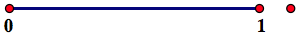Point 3Point 4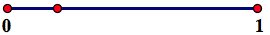Point 5Point 6Point 7Point 8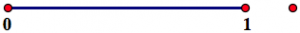Point 9Point 10Point 11Point 12Point 13

Decimals

 (i) 1.20 (ii) $$0. \bar{6}$$ (iii) 0.33 (iv) 0.25 (v) 0.5 (vi) 0.200 (vii) 0.75 (viii) $$0. \bar{3}$$ (ix) 0.2 (x) 1.125 (xi) 0.12 (xii) 0.04 (xiii) 0.40 (xiv) 0.50 (xv) 0.9375

PicturesPicture APicture BPicture CPicture DPicture E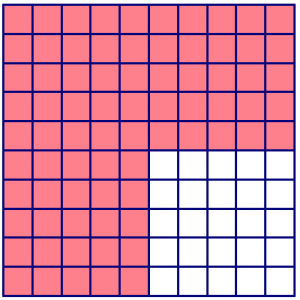Picture FPicture G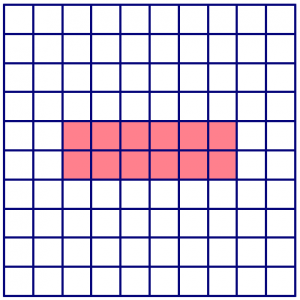Picture HPicture IPicture JPicture KPicture L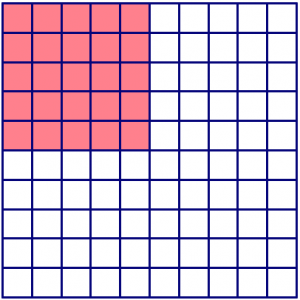Picture M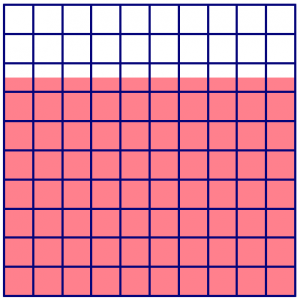Picture NPicture OPicture PPicture Q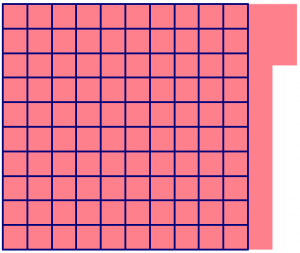Picture R